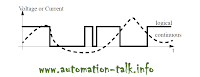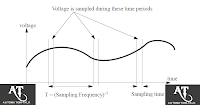## Jun 17, 2011

When we talk of types of Input to PLC , analog input are always seems hard for many PLC programmers. There are basically two types of analog Input for PLC, one is voltage and other is current. In this article we will see that how analog inputs are processed Inside PLC and how PLC Programming of Analog Input is done.

First of all we should understand that an analog value is continuous, not discrete. The type of analog Inputs which we connect to PLC Inputs:
• oven temperature
• fluid pressure
• fluid flow rateWhen we connect any analog input to PLC either current or voltage then it is first sampled by PLC and then converted to a numerical value accordingly by A/D Converter. The sampling of the Value is done at fixed time i.e it has a start and stop time and this value is called "Sampling Time" and inverse of it is known as "Sampling Frequency". Every PLC has its sampling frequency defined on it ,but for a PLC a maximum sampling rate might be 20Hz.The type of analog voltage input which we connect to PLC are generally 0V to 5V, 0V to 10V, -5V to 5V, -10V to 10V. The type of A/D converter in PLC can be 8 bit or 16 bit , if it is 8 bit then it can read 256 different voltage levels.

Now consider an example that we have a 10 bit A/D converter in PLC , so it have resolution of 1024 bits and can read voltages between -10V and 10V , where 0 is -10V and 1023 is +10V. So If a voltage of 4.564V is input into the PLC, the A/D converter converts the voltage to an integer value of 745. When we convert this value back to voltage then it is 4.565V , so a error of +0.001V is there and this is called as quantization error and can be reduced by selecting a higher bit A/D Converter.

So we Hope that you get a Idea on Analog Input Processing in PLC , in the next article we will discuss about the analog outputs in PLC. Till then you can subscribe to get all latest Updates via E mail.Subscribe to Automation-Talk by Email.